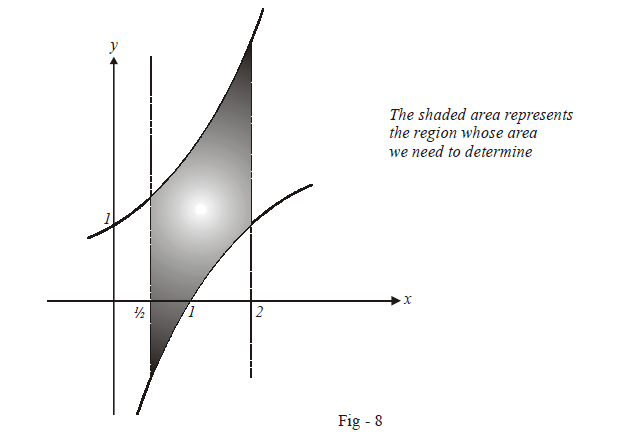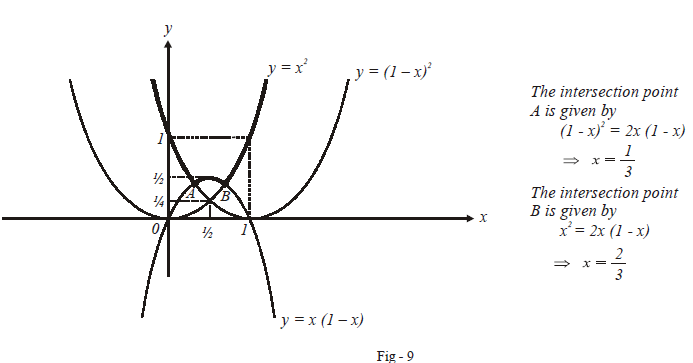# Problems On Areas Set-4

Go back to  'Areas and Volumes'

## Problems On Areas

Example – 7

Find the area of the region bounded by $$x = \frac{1}{2},x = 2,y = 1n\,\,x\,\rm{and}\,y = {2^x}.$$

Solution: The region of interest is verystraightforward to plot:The required area is

\begin{align}A &= \int\limits_{1/2}^2 {\left({{2^x} - 1nx} \right)} dx\\&= \left. {\left( {\frac{{{2^x}}}{{1nx}} -x1n\,x + x} \right)} \right|_{1/2}^2\\&= \left( {\frac{{4 - \sqrt 2}}{{1n\,2}} - \frac{5}{2}1n\,2 + \frac{3}{2}} \right)sq.\,\rm{units}\end{align}

Example – 8

Let $$f\left( x \right)\, =\,\max \left\{ {{x^2},{{\left( {1 - x} \right)}^2},\,2x\,\left( {1 - x}\right)} \right\}.$$ Determine the area of the region bounded by the curve $$y = f\left( x \right),\,x - axis\,,\,x =0\,\rm{and}\,x = 1\,.$$

Solution: The technique to plot the curve for $$f\left( x \right)$$ has been outlined in the unit on Functions. We plot all the three curves $${x^2}$$, $${\left( {1 - x}\right)^2}\,\,\,\rm{and}\,\,\,\,\,2x\left( {1 - x} \right)$$ on the same axes, scan the $$x-$$axis from left to right and at every point, pick out thatgraph which lies uppermost of all the three graphs. In the figure below, theheavyset curve is the curve for $$f\left( x\right):$$We can evaluate the required area, as is clear from thefigure above, by dividing the integration interval [0, 1] into three sub-intervals:

\begin{align}A &= \int\limits_0^{1/3} {{{\left({1 - x} \right)}^2}} dx + \int\limits_{1/3}^{2/3} {2x\left( {1 - x} \right)dx +\int\limits_{2/3}^1 {{x^2}} } \\\\&= \frac{{19}}{{18}} +\frac{{13}}{{81}} + \frac{{19}}{{81}}\,\,\,\,\,\,\,\,\,\,\,\,\,\,\,\,\,\,\,\,\,\,\,\,\,\,\,\,\left(\rm{verify} \right)\\\\&= \frac{{51}}{{81}}\\\\&= \frac{{17}}{{27}}\end{align}